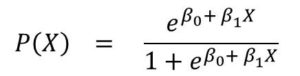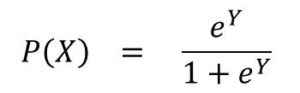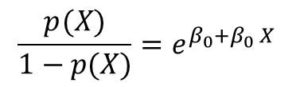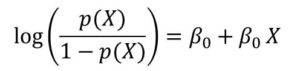# Machine Learning 101 – Basics of Logistic Regression

First I would like clarify that the Logistic Regression model is a model for classification.

Also note that Machine Learning 101 focuses on Supervised Learning. Therefore we always would be discussing Classification and Regression. Machine Learning 102 on the other hand, focuses on Unsupervised Learning (Clustering, Density Estimation and Dimensionality Reduction).

#### What is Logistic Regression?

Logistic regression is a model used when the dependent variable follows a binomial distribution. Simply put, when the variable y, is binary.

And although we would discuss details of probability distribution later, but just know that a distribution is what you get when you plot he probabilities of a random variable against the values of the random variable.

y = f(x)

we say:

p(x) = f(x)

Recall that in linear regression we used a model like this:

p(X) = ß0 + ß1x

In case of logistic regression, we use the logistic function, given as:Don’t worry about how it this function looks. Its quite simple. It’s the same as:where

Y = ß0 + ß1X

The logistic function is good for modelling binary response because, the output of this function would always be between 0 and 1 for all values of X.

#### Odds Ratio

This is a very important concept you should know.

We derive the odds ratio by modifying the logistic function. If we do that, we would have the function below:So the quantity p(X)/[1-p(X)] is called the odds. It can take values from 0 to infinity(∞)

Value of odds close to 0 indicates very low probability while values close to ∞ indicate very high probability.

Odds are sometimes used instead of probability in certain fields. For instance in games.  We can ask: what are the odds of winning this game?  If the probability of winning is let’s say 0.9, then the odds of winning would be 9 – that is 9/(1-9).

Let’s go a little further.

If we take the log of both sides of the odds equation, we would have the equation below:The log expression on the left-hand side is called the logit or log-odds. So we can see that the logistic regression model has a logit that is linear in the variable X.

Unit Change in X

We want to understand the behavior of the logistic regression model. In Logistic regression, increasing value of X by on unit would change the odds by β1 or similarly it multiplies the odds by eβ1.

However, since the relationship between p(X) and X is not linear, β1 does not produce a corresponding change in p(X) associated with a unit increase in X.  The amount that p(X) changes therefore depends on the current value of X.

If  β1  is positive, then increase in X will yield increase in p(X) but if  β1  is negative, then increase in X would result in decrease in p(X). This is irrespective of the value of X.#### kindsonthegenius

Kindson Munonye is currently completing his doctoral program in Software Engineering in Budapest University of Technology and Economics

View all posts by kindsonthegenius →